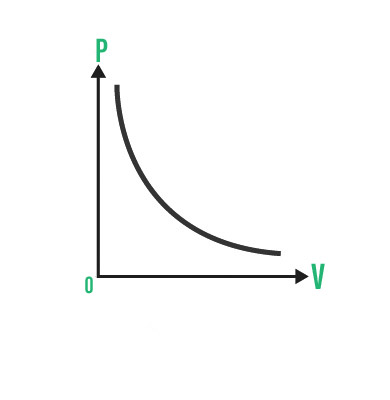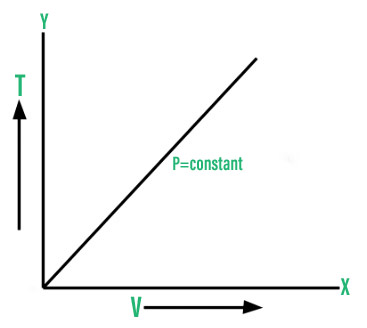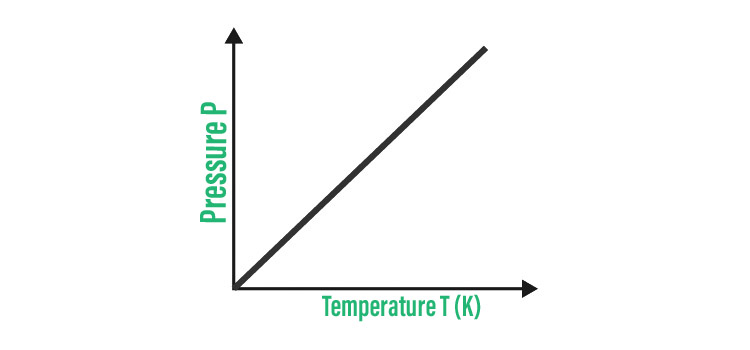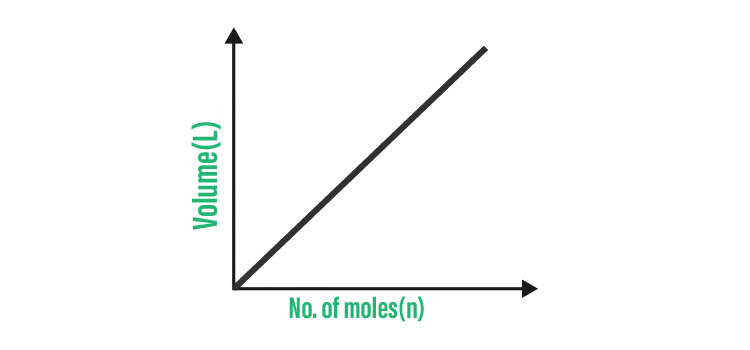GFG App
Open AppBrowser
Continue

# Behavior of Gas Molecules – Kinetic Theory, Boyle’s Law, Charles’s Law

The kinetic theory of gases is a simple, historically significant classical model of gas thermodynamic behavior that laid the groundwork for many fundamental thermodynamic notions. A gas is described by the model as a vast number of identical submicroscopic particles (atoms or molecules) moving in a random, continuous motion. Their size is thought to be substantially smaller than the typical particle distance. The particles collide with one other and with the container’s enclosing walls at random elastic collisions.

The basic form of the model describes an ideal gas and ignores all additional particle interactions. The macroscopic properties of gases, such as volume, pressure, temperature, and transport properties like viscosity, thermal conductivity, and mass diffusivity, are explained by the kinetic theory of gases. Brownian motion and other similar phenomena are also accounted for in the model.

### What is a Gas?

One of the four basic states of matter is gas (the others being solid, liquid, and plasma). A pure gas can be made up of individual atoms such as a noble gas like neon, elemental molecules (such as oxygen, or compound molecules (composed of a variety of atoms) e.g. carbon dioxide. A gas mixture, such as air, consists of several different pure gases. The gaseous state of matter exists between the liquid and plasma phases, with the latter serving as the upper temperature boundary for gases.

Degenerative quantum gases, which exist at the lower end of the temperature scale, are gaining in popularity. High-density atomic gases that have been supercooled to extremely low temperatures are classed as either Bose gases or Fermi gases based on their statistical behavior. See the list of states of matter for a complete list of these unusual states of matter.

The gases are classified as:

• Ideal gas
• Non-ideal gas or real gas

### Kinetic Theory of Gases

The kinetic theory of gases is used to explain the behavior of gas molecules. At the macroscopic level, it is the study of gas molecules.

The five postulates of the kinetic theory of gases are as follows:

• Gas is made up of a vast number of molecules that are constantly moving at random.
• Because the distance between gas molecules is higher than the size of the molecules, the volume of the molecules is negligible.
• Intermolecular interactions are likewise insignificant.
• Molecule collisions with each other and with the container’s walls are always elastomeric.
• The average kinetic energy of all the molecules is depending on the temperature.

### The Factors that Affect the Behavior of Gases-

The following are the factors that influence gas behavior:

1. Temperature (T)
2. Volume (V)
3. Pressure (P)
4. Quantity (n)

The components listed above are interconnected and are listed as follows:

• The volume of the gas expands as the temperature rises due to the expansion of gas molecules.
• Due to the contraction of gas molecules as the temperature drops, the volume of the gas reduces as well.
• Due to the expansion of gas molecules as the temperature rises, the pressure of the gas rises as well.
• Due to the contraction of gas molecules when the temperature drops, the pressure of the gas drops as well.
• The temperature of the gas must be very low or the pressure of the gas must be very high to transform it into solid or liquid.
• When the quantity reduces, the pressure decreases, and when the quantity grows, the pressure increases.
• The volume and amount of the gas should be reduced to reduce pressure.
• To increase pressure, the volume and amount of the gas must be increased.

### The Gas Laws

The following are the five gas laws that govern the behavior of gas molecules:

1. Boyle’s Law

When the amount of gas is fixed at a constant temperature, Boyle’s law states that the volume of the gas is inversely linked to pressure.

P ∝ 1/V

PV = constant

P1V1 = P2V2 = P3V3 = constant

Where,

• P is the pressure of a gas and
• V is the volume of gas.PV graph of Boyle’s law

2. Charles’s Law

The volume of a gas with a fixed mass is linearly proportional to the temperature, according to Charles’ law.

V ∝ T

where

• T is the temperature of a gas, and
• V is the volume of gasT-V graph of Charles’s law

3. Gay-Lussac’s Law

When the volume of the gas remains constant, Gay-law Lussac’s states that the pressure of a given mass of gas varies directly with the absolute temperature of the gas.

P1/T1 = P2/T2

where,

• T1 is the initial temperature,
• P1 is the initial pressure,
• T2 is the final temperature and
• P2 is the final pressure.P-T graph of Gay Lussac’s law

When the pressure and temperature of gas remain constant, Avogadro’s law states that the number of moles and the volume of the gas are proportional.

V ∝ n

or,

V/n = k

where

• V is the volume of the gas,
• n is the number of moles, and
• k is the proportionality constant.### Ideal Gas Law

According to the ideal gas law, the product of pressure and volume of one gram molecule is equal to the product of the number of moles of the gas, the universal gas constant, and the absolute temperature.

PV = nRT

where,

• P is the pressure of the gas,
• V is the volume of the gas,
• n is the number of moles,
• R is the universal gas constant = 8.3145 J.mol-1.K-1,
• T is the temperature of the gas, and
• N is Avogadro’s number, NA = 6.0221×1023

Ideal Gas Properties and Characteristics

1. Ideal gas motion along a straight line is both constant and random.
2. Because the particles in the gas are so minute, the gas takes up very little space.
3. There is no interaction between the gas particles. Particles only collide automatically with the container’s walls and each other.
4. The absolute temperature is directly proportional to the average kinetic energy of the gas particle.
5. Many of the same particles (atoms or molecules) make up the gases, which are perfectly rigid spheres that are also incredibly small.
6. Because the volume of a gas molecule is so small in comparison to the space between them, they are referred to as point masses.

### Sample Problems

Problem 1: At 3.500 atm, an 18.10mL sample of gas is taken. What will the volume be if the pressure rises to 2.500 atm and the amount of gas and temperature remain constant?

Solution:

Boyle’s law equation is used to solve the problem.

P1V1 = P2V2

V2 = P1V1 / P2

V2 = (18.10 * 3.500atm)/2.500atm

V2 = 25.34 mL

Problem 2: A carbon dioxide sample in a pump has a volume of 21.5 mL and is at a temperature of 50.0 C. Find the new volume of carbon dioxide in the pump if the temperature is raised to 75.0 C while the amount of gas and pressure stays constant.

Solution:

Using Charles’s law

V2 = V1T2/T1

V2 = 7,485.225/ 323.15

V2 = 23.16 mL

Problem 3: Calculate the change in pressure when a constant volume of gas at 2.00 atm is heated from 30.0 to 40.0 degrees Celsius.

Solution:

Gay-Lussac law is used to solve this problem;

P1T1 = P2T2

P2 = 626.3/303.15

P2 = 2.0659

Problem 4: 6.00 L of gas contains 0.975 mol at constant temperature and pressure. What additional volume will arise if the amount of gas is increased to 1.90 mol?

Solution:

V1n1 = V2n2

V2 = (6*1.90)/0.975

V2=  11.69L

Problem 5: A sealed jar with a volume of exactly 1L and 1 mole of air at 20 degrees Celsius, assuming that the air behaves like an ideal gas. So, in Pa, how much pressure is inside the jar?

Solution:

By solving with the help of ideal gas equation;

PV=nRT

By rearranging the equation we can get,

P=nRT/V

Write down all the values which are known in S.I unit

n= 1

R= 8.314J/K/mol

T= 20degree Celsius=(20+273.15)K=293.15K

V=1L=0.001m3

Put all the values in the equation

P= nRT/V

P=(1*8.314*293.15)/0.001

P= 2,437,249

P=2.437*10^6 Pa

The pressure is almost 24atm.

Problem 6: What are the factors which affect the behavior of gases?

Solution:

The following are the factors that influence gas behavior:

1. Temperature (T)
2. Volume (V)
3. Pressure (P)
4. Quantity (n)

The behavior of gas molecules is explained by the kinetic theory of gases, which is a fundamental concept in physics and chemistry. The kinetic theory of gases describes the behavior of gas molecules in terms of their motion and collisions, and it is based on a number of key assumptions:

Gas molecules are in constant random motion, traveling in straight lines and changing direction only when they collide with other molecules or with the walls of their container.

1. Gas molecules are extremely small compared to the distances between them, and so their volume is negligible compared to the volume of their container.
2. Gas molecules do not attract or repel each other, except during collisions.
3. The collisions between gas molecules and with the walls of their container are perfectly elastic, meaning that no energy is lost during the collision.
4. The temperature of a gas is proportional to the average kinetic energy of its molecules.

Based on these assumptions, the behavior of gases can be described by a number of laws, including Boyle’s law and Charles’s law.

1. Boyle’s law states that at constant temperature, the pressure of a gas is inversely proportional to its volume. In other words, if the volume of a gas is decreased, its pressure will increase, and vice versa. This law can be explained by the kinetic theory of gases, which shows that when the volume of a gas is decreased, the molecules are forced closer together, leading to more frequent and energetic collisions with the walls of the container, which results in a higher pressure.
2. Charles’s law states that at constant pressure, the volume of a gas is directly proportional to its temperature. In other words, if the temperature of a gas is increased, its volume will also increase, and vice versa. This law can be explained by the kinetic theory of gases, which shows that when the temperature of a gas is increased, the molecules will move faster and collide more frequently with the walls of the container, leading to a larger volume.
3. Overall, the kinetic theory of gases provides a powerful framework for understanding the behavior of gas molecules, and it has important applications in many areas of science and technology, including thermodynamics, fluid mechanics, and atmospheric science.

My Personal Notes arrow_drop_up